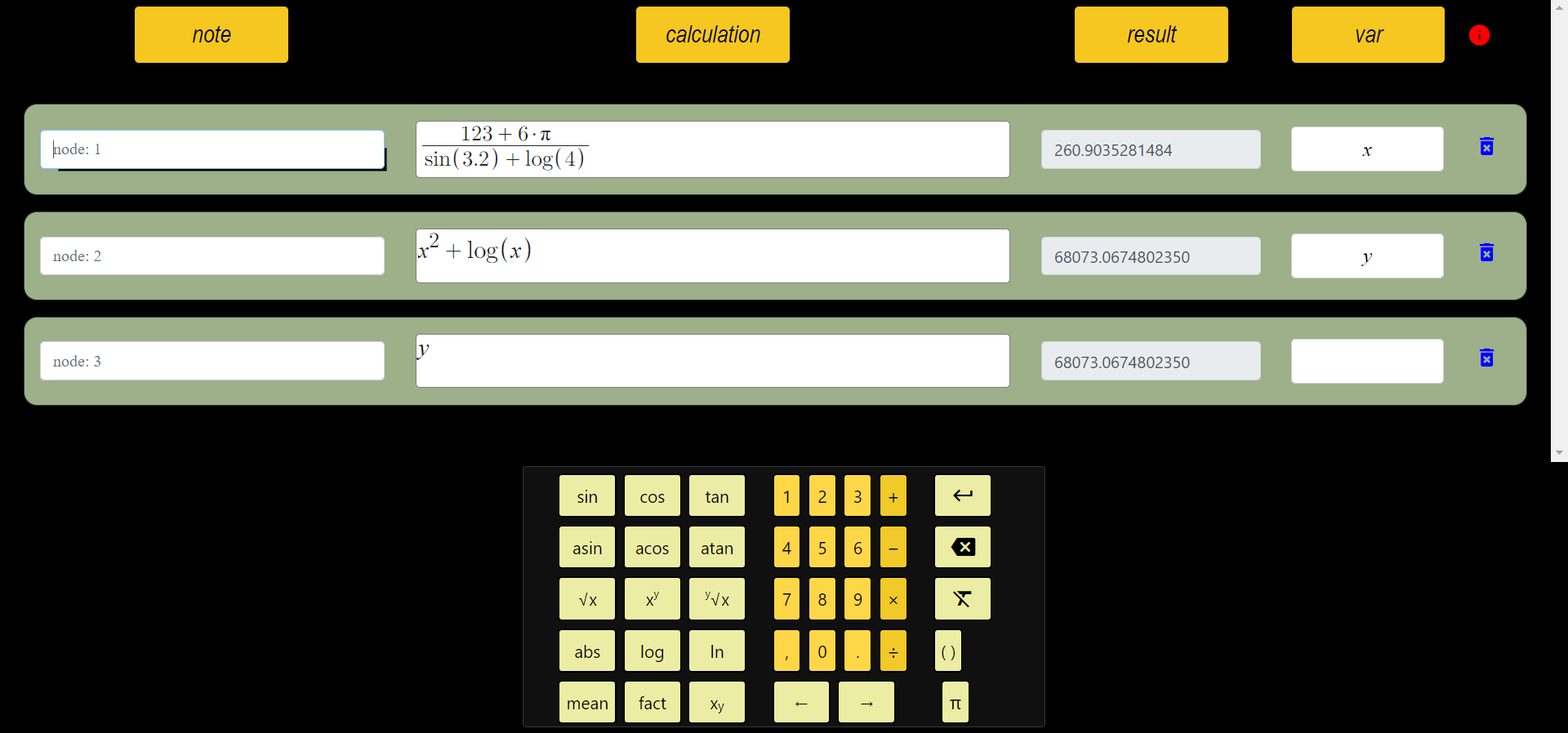# What is function?

A function is a rule or formula that binds each element in set X (the definition) to exactly one and only one element in set Y (image). Not every element in Y has to be an output, and different elements in X can have the same output. A rule that assigns each element x in X to exactly one element y in Y is called a function f from a set of X elements to a set of Y elements. A function can also be thought of as a collection of ordered pairs (x, y). For every y-value in the set, there is only one x-value "but different x's can give the same y". This indicates that no x value with different y values ​​can appear more than once. To define a function in this context, we typically use the rule y = f(x) and then plot the ordered pairs (x, f(x)) in the Cartesian Plane to produce a graph of that function. Using this graphical representation, we can use a test to determine whether we have a graph of a function: The Vertical Line Test is a method for determining whether an equation is a graph. But before that, it will be useful to look at the basic properties of functions.

## Dimension set of function

For a function f(x)=y, let the domain of f be the set X. When we express a function as a collection of ordered pairs (x, y), these x values ​​correspond to the domain. If only the rule y = f(x) is given, the domain is taken as the set of all real x's for which the function is defined. Value Set of the Function f: The value set of the function X -> Y is the set of y values ​​that provide y = f(x) for some x in X. When we express a function as a collection of ordered pairs (x, y), these y values ​​correspond to the set of values.

## Graphical Behavior of Functions

As part of our investigation of how functions change, we can identify intervals where the function changes in certain ways. If function values ​​get larger as input values ​​increase within a range, we say that the function increases in that range. Similarly, if function values ​​decrease as input values ​​increase across an interval, the function decreases over that interval.

### Vertical Line Test

According to the Vertical Line Test, if you cannot draw a vertical line that intersects a graph at more than one point (green), the graph is a function. Otherwise it is not a function (red):###### All Articles

id: Sjgsp8LU0x